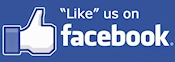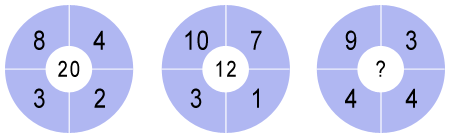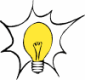# Number Puzzle #16- Number In The Middle -

In the following circles, the four outer numbers are used to calculate the number in the middle. Can you figure out the rule to determine what number should replace the question mark in the third circle?Need a Hint?